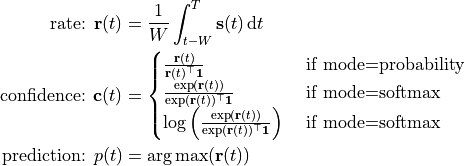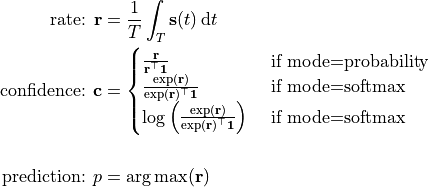# Classifier

Classifier modules.

class lava.lib.dl.slayer.classifier.MovingWindow(time_window, mode='probability', eps=1e-06)

Moving window based classifier. It produces a timeseries of classification/prediction based on moving window estimate.Parameters
• time_window (int) – size of moving window.

• mode (str) – confidence mode. One of ‘probability’|’softmax’|’logsoftmax’. Defaults to ‘probability’.

• eps (float) – infinitesimal value. Defaults to 1e-6.

Examples

>>> classifier = MovingWindow(20)
>>> prediction = classifier(spike)

confidence(spike, mode=None)

Moving window confidence.

Parameters
• spike (torch tensor) – spike input.

• mode (str) – confidence mode. If it is None, the object’s mode is used. Defaults to None.

Returns

output confidence.

Return type

torch tensor

Examples

>>> confidence = classifier.confidence(spike)

forward(spike)
predict(spike)

Moving window prediction.

Parameters

spike (torch tensor) – spike input.

Returns

output prediction.

Return type

torch tensor

Examples

>>> prediction = classifier.predict(spike)

rate(spike)

Moving window spike rate.

Parameters

spike (torch tensor) – spike input.

Returns

spike rate.

Return type

torch tensor

Examples

>>> rate = classifier.rate(spike)

class lava.lib.dl.slayer.classifier.Rate

Global rate based classifier. It considers the event rate of the spike train over the entire duration as the confidence score.Examples

>>> classifier = Rate
>>> prediction = classifier(spike)

static confidence(spike, mode='probability', eps=1e-06)

Given spike train, returns the confidence of the output class based on spike rate.

Parameters
• spike (torch tensor) – spike tensor. First dimension is assumed to be batch, and last dimension is assumed to be time. Spatial dimensions are collapsed by default.

• mode (str) – confidence mode. One of ‘probability’|’softmax’|’logsoftmax’. Defaults to ‘probability’.

• eps (float) – infinitesimal value. Defaults to 1e-6.

Returns

confidence.

Return type

torch tensor

Examples

>>> confidence = classifier.confidence(spike)
>>> confidence = Rate.confidence(spike)

forward(spike)
static predict(spike)

Given spike train, predicts the output class based on spike rate.

Parameters

spike (torch tensor) – spike tensor. First dimension is assumed to be batch, and last dimension is assumed to be time. Spatial dimensions are collapsed by default.

Returns

indices of max spike activity.

Return type

torch tensor

Examples

>>> prediction = classifier.predict(spike)
>>> prediction = Rate.predict(spike)

static rate(spike)

Given spike train, returns the output spike rate.

Parameters

spike (torch tensor) – spike tensor. First dimension is assumed to be batch, and last dimension is assumed to be time. Spatial dimensions are collapsed by default.

Returns

spike rate.

Return type

torch tensor

Examples

>>> rate = classifier.rate(spike)
>>> rate = Rate.rate(spike)